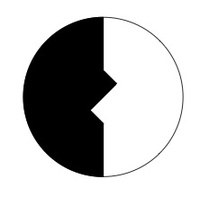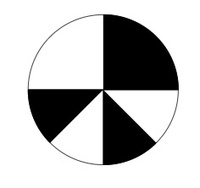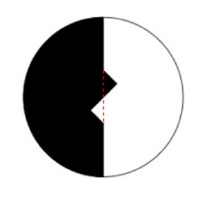Representing Half of a Circle

Alignments to Content Standards: 3.G.A.2

For each picture, decide whether one half of the circle is shaded or not. Explain how you know.

1.2.3.4.Solutions

Solution: 1

1. This picture represents one half. The shaded area is equal in size and shape to the unshaded area: this can be shown by rotating the picture, about the center of the circle, by $180$ degrees so that the black and white parts trade places. Alternatively, if the shape is cut, the white part is the same shape and size as the black part.

2. This picture does not represent one half. The shaded part is visually larger than the unshaded part. Cutting out the unshaded pieces will show that the unshaded part is in fact equal to one of the two equal shaded pieces so that the shaded part actually represents two thirds of the circle.

3. This picture represents $\frac{2}{4}$ as there are four equally sized pieces and two of them are shaded. Since $\frac{2}{4}$ is equivalent to $\frac{1}{2}$ the shaded portion of this picture does represent one half. Another way to see this would be to cut out the two shaded and unshaded pieces and observe that each shaded piece is identical in shape and size to an unshaded piece making the shaded and unshaded areas equal.

4. This picture is a creative and interesting way to represent one half of a circle. To see that the shaded part represents one half of the circle, notice that this picture becomes identical to picture (c) if the lower right shaded slice of the circle is moved over next to the lower left shaded slice. Alternatively, the picture can be rotated counterclockwise by $90$ degrees and the shaded and unshaded parts trade places and so they must be equal. Thirdly, we can observe that each shaded piece may be paired up with an unshaded piece of equal size so again the shaded and unshaded areas must be equal, meaning that the shaded area represents one half of the whole circle.

Solution: Student solution

a. If I imagine a line through the center like the one below, then I can see two small triangles that are the same size and shape. If the shaded and unshaded triangles switch places, I can see that half the circle is shaded.b. If I imagine moving the two unshaded pieces together, I can see that they don't fill up half the circle. So more than half must be shaded.

c. The circle is in 4 equal parts and 2 are shaded. So half the circle is shaded.

d. The small shaded piece on the left is the same size as the unshaded piece on the right. I imagined that those two pieces traded places, so that I could see that half the circle is shaded.